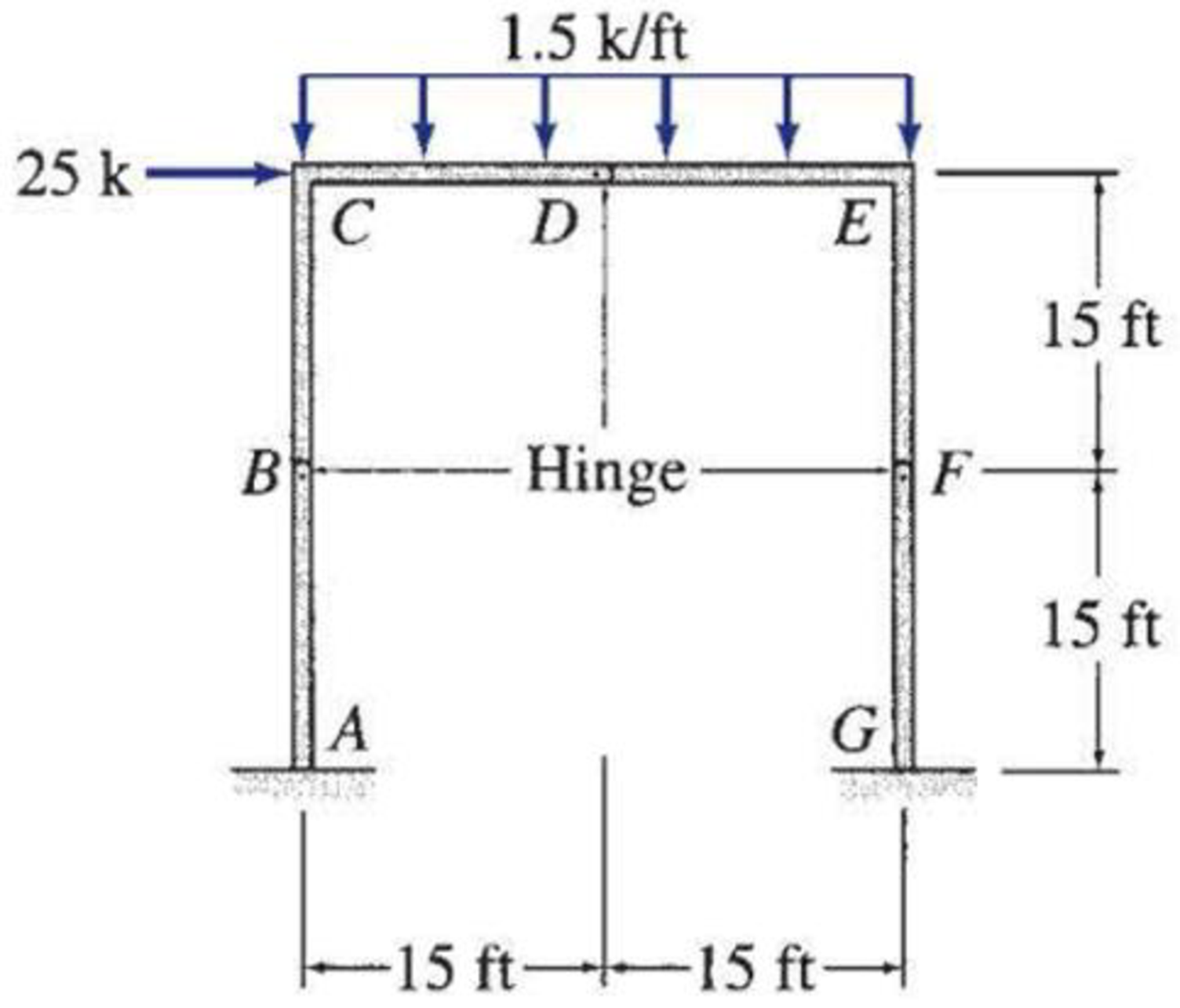# 5.57 through 5.71 Draw the shear, bending moment, and axial force diagrams and the qualitative deflected shape for the frame shown.

#### Solutions

Chapter
Section
Chapter 5, Problem 71P
Textbook Problem
18 views

## 5.57 through 5.71 Draw the shear, bending moment, and axial force diagrams and the qualitative deflected shape for the frame shown.To determine

Plot the shear diagram, bending moment diagram, axial force diagram, and the qualitative deflected shape of the frame.

### Explanation of Solution

Write the condition for static instability, determinacy and indeterminacy of plane frames as follows:

3m+r<3j+ecstaticallyunstableframe        (1)

3m+r=3j+ecstaticallydeterminateframe        (2)

3m+r>3j+ecstaticallyindeterminateframe        (3)

Here, number of members is m, number of external reactions is r, the number of joints is j, and the number of elastic hinges is ec.

Find the degree of static indeterminacy (i) using the equation;

i=(3m+r)(3j+ec)        (4)

Refer to the Figure in the question;

The number of members (m) is 3.

The number of external reactions (r) is 6.

The number of joints (j) is 4.

The number of elastic hinges ec is 3.

Substitute the values in Equation (2);

3(3)+6=3(4)+315=15staticallydeterminateframe

Show the free-body diagram of the entire frame as in Figure 1.

Refer Figure 1,

Consider the section BCDEFG:

+MBBCDEFG=025(15)1.5(30)(302)Gx(15)+Gy(30)+MG=037567515Gx+30Gy+MG=015Gx+30Gy+MG=1050        (1)

Consider the section DEFG:

+MDDEFG=01.5(15)(152)Gx(30)+Gy(15)+MG=0168.7530Gx+15Gy+MG=030Gx+15Gy+MG=168.75        (2)

Consider the section FG:

+MDFG=0Gx(15)+MG=015Gx+MG=0        (3)

Solve the Equations (1), (2), and (3) simultaneously.

Gx=23.75k

Gy=35k

MG=356.25k-ft

Consider entire frame:

Find the vertical reaction at point A by resolving the vertical component of forces.

+Fy=0Ay1.5(30)+Gy=0Ay45+35=0Ay=10k

Find the moment at point A by taking moment about point A.

+MA=0MA25(30)1.5(30)(302)+MG+Gy(30)=0MA750675+356.25+35(30)=0MA=18.75k-ft

Find the horizontal reaction at point A by resolving the horizontal component of forces.

+Fx=0Ax+25Gx=0Ax+2523.75=0Ax=1.25k

Show the free-body diagram of the members and joints of the entire frame as in Figure 2.

Consider point A:

Resolve the vertical component of forces.

+FY=010AYAC=0AYAC=10k

Resolve the horizontal component of forces.

+FX=0AXAC1.25=0AXAC=1.25k

Take moment about the point A.

+MAAC=018.75MAAC=0MAAC=18.75k-ft

Consider the member AC:

Resolve the vertical component of forces.

+FY=0AYACCYAC=010CYAC=0CYAC=10k

Resolve the horizontal component of forces.

+FX=0AXAC+CXAC=01

### Still sussing out bartleby?

Check out a sample textbook solution.

See a sample solution

#### The Solution to Your Study Problems

Bartleby provides explanations to thousands of textbook problems written by our experts, many with advanced degrees!

Get Started

Find more solutions based on key concepts
Identify and list at least ten different materials that are used in a car.

Engineering Fundamentals: An Introduction to Engineering (MindTap Course List)

What are some common information systems used by functional units in a typical enterprise? (555)

Enhanced Discovering Computers 2017 (Shelly Cashman Series) (MindTap Course List)

Explain the differences among data, information, and a database.

Database Systems: Design, Implementation, & Management

What is the purpose of a typical inverter?

Automotive Technology: A Systems Approach (MindTap Course List)

We use lots of codes in our personal and business lives. How many can yon class name?

Systems Analysis and Design (Shelly Cashman Series) (MindTap Course List)

Why should you never change the current setting during a weld?

Welding: Principles and Applications (MindTap Course List)

If your motherboard supports ECC DDR3 memory, can you substitute non-ECC DDR3 memory?

A+ Guide to Hardware (Standalone Book) (MindTap Course List)